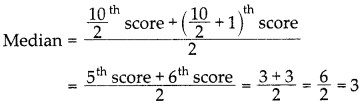# DAV Class 7 Maths Chapter 12 Worksheet 2 Solutions

The DAV Maths Class 7 Solutions and DAV Class 7 Maths Chapter 12 Worksheet 2 Solutions of Data Handling offer comprehensive answers to textbook questions.

## DAV Class 7 Maths Ch 12 WS 2 Solutions

Question 1.
Find the median of the following data.
11, 39, 43, 45, 25, 46, 43, 42, 37.
Let us arrange the given data in ascending order,
11, 25, 37, 39, 42, 43, 43, 45, 46
Here number of observations = 9 (odd)
∴ Median = $$\frac{9+1}{2}$$ = 5th observation = 42
Hence the median = 42.

Question 2.
Find the median of.
(i) 85, 42, 56, 69, 51, 81, 72, 92, 67
(ii) 15, 47, 48, 81, 17, 27, 9, 3, 10, 75
(i) Let us arrange the given data in ascending order,
42, 51, 56, 67, 69, 72, 81, 85, 92
Number of observations = 9 (odd)
Median = $$\frac{9+1}{2}$$ = 5th observation = 69
Hence the median = 69.

(ii) Let us arrange the given observations in ascending order,
3, 9, 10,15,17, 27, 47, 48, 75, 81
Number of observations = 10 (even)
∴ Median = $$\frac{\frac{10^{\text {th }}}{2} \text { observation }+\left(\frac{10}{2}+1\right)^{\text {th }} \text { observation }}{2}$$
= $$\frac{5^{\text {th }} \text { observation }+6^{\text {th }} \text { observation }}{2}=\frac{17+27}{2}=\frac{44}{2}$$ = 22
Hence the median = 22.Question 3.
The following number of goals were scored by a team in a series of 10 matches:
2, 3, 5, 4, 0, 1, 3, 3, 3, 4
Find the median of these scores.
Arranging the given scores in increasing order
0,1, 2, 3, 3, 3, 3, 4, 4, 5
Number of scores = 10 (even)Hence the median = 3.

Question 4.
The weight (in kg) of 11 students of class -VII (in April 2015) is 42, 52, 48, 52, 55, 58, 53, 54, 46, 49, 57
Find the median of these weights. In July 2015, one student of weight 58 kg, left the school and a new student of weight 68 kg joined the class. Find new median.
Median = $$\frac{11+1}{2}=\frac{12}{2}$$ = 6th observation = 52